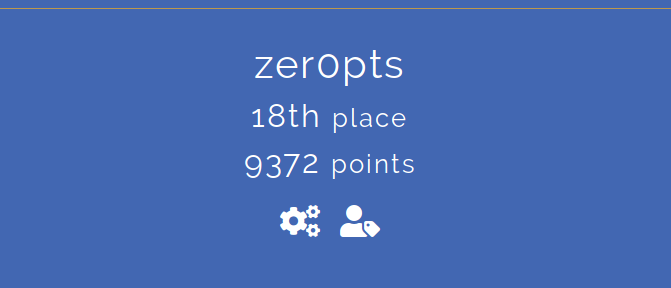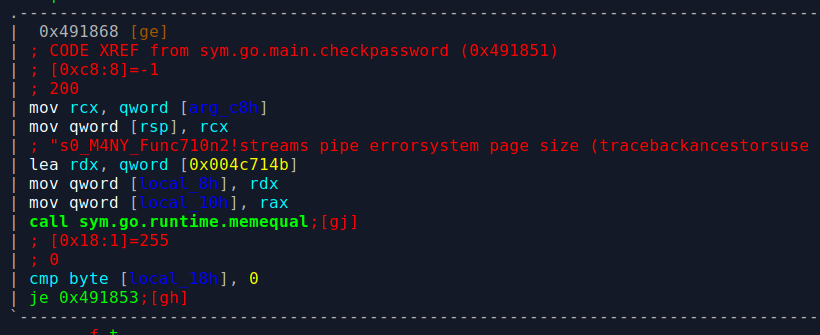# ふるつき

## v(*'='*)v かにI played Facebook CTF as a member of team `zer0pts`. We were in 18th place and got 9372 points. There were a lot of well-crafted challenges. I enjoyed all the challenges I tried even though I couldn't solve some of them.

## [Crypto 453points (79 solves)]keybaseish

We built a secure messaging platform based on twitter identities, verified with our proprietary signature protocol! Right now our founder is the only one using it. Would you like to join?

Note: You do not need a twitter account to solve this challenge

http://challenges.fbctf.com:8080

This site used a unique way to recover the account. The user tweets the RSA encrypted PIN value (`pow(pin, e, n)` in his own twitter account and upload the encryption key (`e` and `n`). If the tweeted ciphervalue is match to the server-side encryption the account will be recovered.

The administrator has already tweeted a ciphervalue in past as shown below.

`43522081190908620239526125376626925272670879862906206214798620592212761409287968319160030205818706732092664958217053982767385296720310547463903001181881966554081621263332073144333148831108871059921677679366681345909190184917461295644569942753755984548017839561073991169528773602380297241266112083733072690367`

We just did a search for the appropriate e and n of the given PIN value. I used the following script.

```from Crypto.PublicKey import RSA
from Crypto.Util.number import getPrime
from Crypto import Random

RSAkey = RSA.generate(1024)
key_params = RSAkey.__getstate__()
sign = 43522081190908620239526125376626925272670879862906206214798620592212761409287968319160030205818706732092664958217053982767385296720310547463903001181881966554081621263332073144333148831108871059921677679366681345909190184917461295644569942753755984548017839561073991169528773602380297241266112083733072690367

pin = int(input().strip())
n = getPrime(pin.bit_length() + 1)
for i in range(2, n):
x = pow(sign, i, n)
if x == pin:
print(x)
print("{}:{}".format(i, n))
exit()
```

If we choose a prime as modulo, it forms a cyclic group*1. Thus, it will be a correct `e` in [1, n).

## [Reversing 453points (79 solves)] go_get_the_flag

Go get the flag!

We were given an ELF file which is written in go. Just open it in `radare2`, and see `checkpassword` function. We could see the specious string in it: s0_M4NY_Func710n2!`.I ran `./ggtf s0_M4NY_Func710n2!` then got the flag: `fb{.60pcln74b_15_4w350m3}`.

## [Reversing 738points (55 solves)]matryoshka

There was a downloader found on a Mac desktop. It's your job to have layers of fun getting the flag.

Written by malwareunicorn

I solved first three stages. My teammate @ptr-yudai solved the last stage and got the flag.

We were given an executable which runs on the macOS. It was multi staged crackme challenge, but downloaded a corrupted PNG image before running as a crackme. The downloaded image includes some machine codes. The binary ran them.

On the first stage, the machine code is located at `393216` in the image. I used `cutter` to reversing it. Then I found that the code did rot13 to the input and compared it to `4ZberYriryf2TbXrrcTbvat`. Then the first value is `4MoreLevels2GoKeepGoing`.

The 2nd, 3rd and 4th stages were countinuoused. The machine code is located at `424056`. It used an input to decrypt next stage's machine code.

The second stage required 4 bytes as input, and subtracted it from encrypted machine code. There was a check if the decrypted machine code starts with `0x55488945` (`push rbp; mov rbp rsp;`). Thus, to get the keyword, we can compare encrypted machine code and `0x55488945`. The following script told me that the key value is `r00t`.

```r13 = [0x59, 0xb9, 0x78, 0xc7]
correct = [0xe5, 0x89, 0x48, 0x55]
ans = [[] for _ in range(4)]

for p in range(4):
for c in range(256):
if (r13[p] - c + 256) % 256 == correct[p]:
ans[p].append(chr(c))
print(ans)
```

Now we can decrypt the machine code of the third stage.

```offset = 0x137
size = 0x678

data = open("code2", "rb").read()[offset : offset + size]
key = [ord(c) for c in "r00t"]

new_opecodes = []

for i in range(0, size):
new_opecodes.append((data[i] - key[i % 4] + 256) % 256)
open("stage3_code", "wb").write(bytearray(new_opecodes))
```

The stage3 was simple xor. Since it also checks the function header, we can get the 4 bytes of key value easily. However, in this stage, it required 8 bytes key. Luckily, latter bytes was determined to be `uOQJ` because there was a raw-compare in the code.

```r15 = [0x19, 0x02, 0xea, 0x87][::-1]
correct = [0xe5, 0x89, 0x48, 0x55]
ans = []

for i in range(4):
ans.append(chr(r15[i] ^ correct[i]))

ans = "".join(ans)[::-1] + "uOQJ"
print(ans)
```

The third keyword was `LJcbuOQJ`. We can extract 4th stage's machine code.

```offset = 0x12c
size = 0x44a

data = open("stage3_code", "rb").read()[offset : offset + size]
key = [ord(c) for c in "LJcbuOQJ"]

new_opecodes = []

for i in range(0, size):
new_opecodes.append(data[i] ^ key[i % 8])
open("stage4_code", "wb").write(bytearray(new_opecodes))
```

The last part was pretty hard. It also required 8bytes value and the last 4 bytes was `wT96`, but first 4 bytes can be any letters. As the input value is used for some calculation to get the return value and a string, this process may help us determine the first bytes.

However, I completely stucked here. Then my teammate @ptr-yudai wrote a bruteforce script and got the correct input: `YrQmwT96`.

```#include <stdio.h>
#include <string.h>
#include <stdlib.h>
#include <byteswap.h>

unsigned char memory[0x100];
unsigned char original[0x30] =
"\xf6\x2c\x72\x1a\x03\x99\x0e\x78\xbd\x90\xe9\x68\xd0\x69\x37\x29"
"\xf8\x12\xf4\xe5\xd0\xfb\xf3\x7e\x72\x61\x79\x19\xed\x44\x12\x52"
"\xf5\xf9\xaa\x14\x36\x0d\x1f\xb2\x52\x6b\xf2\x6a\xda\x9d\xec\x3c";
unsigned char string[0x30];

{
unsigned long delta = 0x0808080808080808;
unsigned long seed = 0x0706050403020100;
unsigned long *ptr = (unsigned long*)&memory;
int i;
for(i = 0; i < 0x20; i++) {
*ptr = seed;
seed += delta;
ptr++;
}
unsigned char r9 = 0, r10 = 0;
for(i = 0; i < 0x100; i++) {
unsigned char xmm0 = memory[i];
memory[i] = memory[r9];
memory[r9] = xmm0;
r10 = (r10 + 1) % 8;
}
}

void create_string()
{
int i;
unsigned char pos0 = 0, pos1 = 0;
for(i = 0; i < 0x30; i++) {
pos0 += 1;
unsigned char xmm2 = memory[pos0];
pos1 += xmm2;
unsigned char xmm3 = memory[pos1];
memory[pos0] = xmm3;
memory[pos1] = xmm2;
string[i] ^= memory[(xmm2 + xmm3) & 0xff];
}
}

int main()
{
char c1, c2, c3, c4;
for(c1 = 0x20; c1 < 0x7f; c1++) {
printf("c1 = %d\n", c1);
for(c2 = 0x20; c2 < 0x7f; c2++) {
for(c3 = 0x20; c3 < 0x7f; c3++) {
for(c4 = 0x20; c4 < 0x7f; c4++) {
memcpy(string, original, 0x30);

create_string();

unsigned long ret = *(unsigned long*)&(string);
unsigned char *text = (unsigned char*)((unsigned long)string + 8);

int i;
for(i = 0; i < 0x28; i++) {
if (!(0x20 <= text[i] && text[i] < 0x7f)) {
break;
}
}
if (i > 0x20) {
printf("ret: %016lx\n", ret);
printf("text: %s\n", text);

unsigned long d, c, b, a;
unsigned long x = __bswap_64(ret);
d = 0x115c28da834feffd ^ x;
c = 0x665f336b1a566b19 ^ d;
b = 0x393b415f5a590044 ^ c;
a = 0x3255557376f68 ^ b;
printf("%016lx %016lx %016lx %016lx\n", a, b, c, d);
}
}
}
}
}
return 0;
}
```